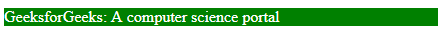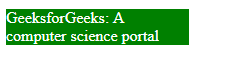Related Articles

# CSS | max-width Property

• Last Updated : 03 Jan, 2019

The max-width property in CSS is used to define the maximum width of an element. The value of the width cannot be larger than the value by max-width. If the content is larger then the max-width then it will go to the next line and if the content is smaller then max-width then it has no effect.

Syntax:

`max-width: none|length|initial|inherit;`

Property Values:

• none: It is the default value and it does not contains max-width.

Syntax:

`max-width: none;`

Example:

 ```<``html``>``    ``<``head``>``        ``<``title``>max-width property``         ` `        ````        ``<``style``>``            ``p {``                ``max-width: none;``                ``color:white;``                ``background-color:green;``            ``}``        ````    ````     ` `    ``<``body``>``        ``<``p``>``            ``GeeksforGeeks: A computer science portal``        ````    ````                    `

Output:• length: This property is used to set the length of max-width. The length can be set in form of px, cm etc.

Syntax:

`max-width: length;`

Example:

 ```<``html``>``    ``<``head``>``        ``<``title``>max-width property``         ` `        ````        ``<``style``>``            ``p {``                ``max-width: 110px;``                ``color:white;``                ``background-color:green;``            ``}``        ````    ````     ` `    ``<``body``>``        ``<``p``>``            ``GeeksforGeeks: A computer science portal``        ````    ````                    `

Output:• percentage (%): This property is used to set the max-width in form of percentage.

Syntax:

`max-width: %;`

Example:

 ```<``html``>``    ``<``head``>``        ``<``title``>max-width property``         ` `        ````        ``<``style``>``            ``p {``                ``max-width: 20%;``                ``color:white;``                ``background-color:green;``            ``}``        ````    ````     ` `    ``<``body``>``        ``<``p``>``            ``GeeksforGeeks: A computer science portal``        ````    ````                    `

Output:• initial: It is used to set max-width property to its default value.

Syntax:

`max-width: initial;`

Example:

 ```<``html``>``    ``<``head``>``        ``<``title``>max-width property``         ` `        ````        ``<``style``>``            ``p {``                ``max-width: initial;``                ``color:white;``                ``background-color:green;``            ``}``        ````    ````     ` `    ``<``body``>``        ``<``p``>``            ``GeeksforGeeks: A computer science portal``        ````    ````                    `

Output:• inherit: This property is inherited from its parent.

Supported Browsers: The browser supported by max-width property are listed below: